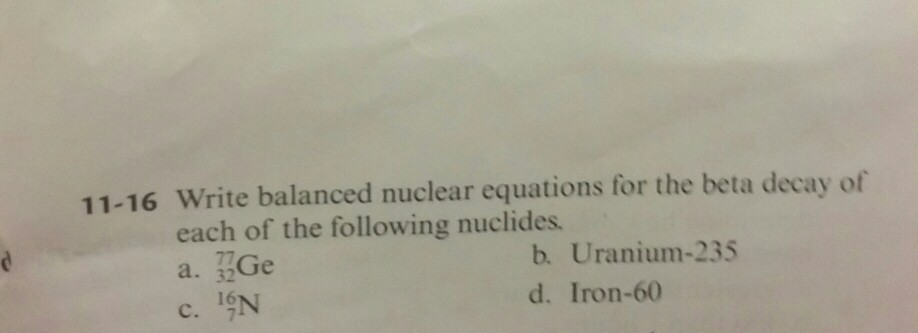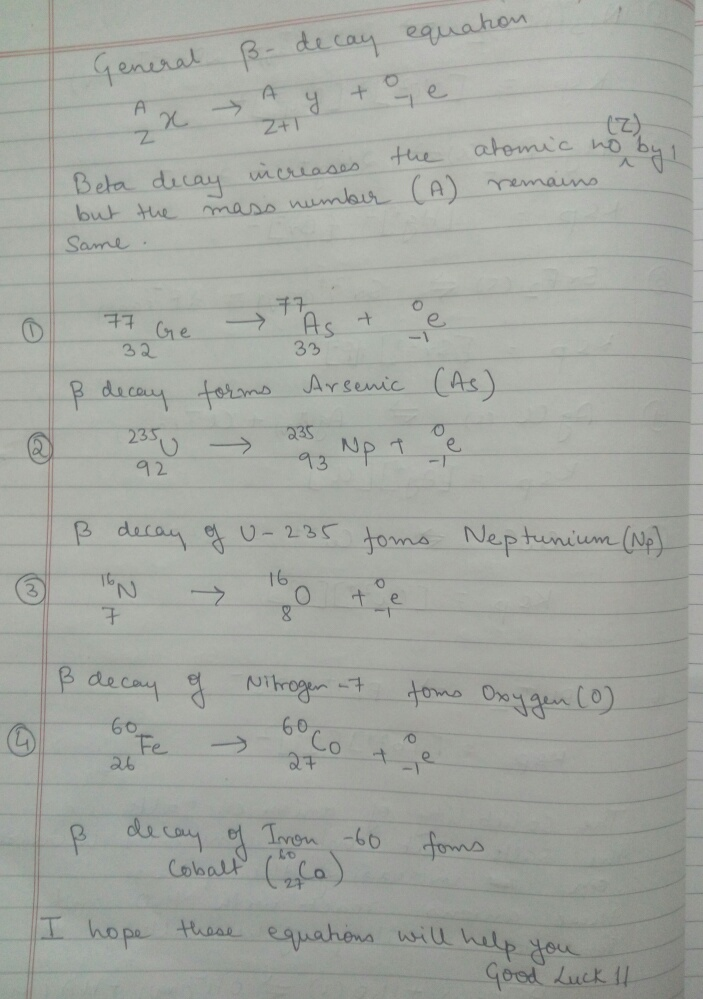# Question & Answer: Write balanced nuclear equations for the beta decay of each of the following nuclides. a……Write balanced nuclear equations for the beta decay of each of the following nuclides. a. ^77_32 Ge b. Uranium – 235 c. ^16_7 N d. Iron -60